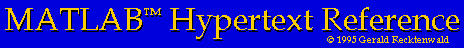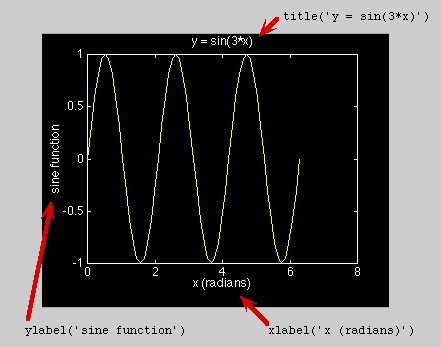# Creating Line Plots with MATLAB

This page describes how to plot y = f(x) by connecting the data points with a line.

## The basic plot command

Two-dimensional line and symbol plots are created with the plot command. In its simplest form plot takes two arguments
```	>> plot(xdata,ydata)
```
where xdata and ydata are vectors containing the data. Note that xdata and ydata must be the same length and both must be the same type, i.e., both must be either row or column vectors. Additional arguments to the plot command provide other options including the ability to plot multiple data sets, and a choice of colors, symbols and line types for the data.

### A simple line plot

Here are the MATLAB commands to create a simple plot of y = sin(3*pi*x) from 0 to 2*pi. For your convenience these commands are also stored in the sin3xPlot.m script file. (Refer to ``help with downloading scripts'' for instructions on downloading this script to your computer.)
```	>> x = 0:pi/30:2*pi;         %  x vector, 0 <= x <= 2*pi, increments of pi/30
>> y = sin(3*x);             %  vector of y values
>> plot(x,y)                 %  create the plot
>> xlabel('x (radians)');    %  label the x-axis
>> ylabel('sine function');  %  label the y-axis
>> title('sin(3*x)');        %  put a title on the plot
```

The effect of the labeling commands, xlabel, ylabel, and title are indicated by the text and red arrows in the figure. Don't skimp on the labels!### Logarithmic axis scaling

Log-log and semi-log plots are created with commands that act just like the plot command. These are summarized in the table below

Command Name Plot type
loglog log(y) versus log(x)
semilogx y versus log(x)
semilogy log(y) versus x
Commands for creating log-log and semilog plots